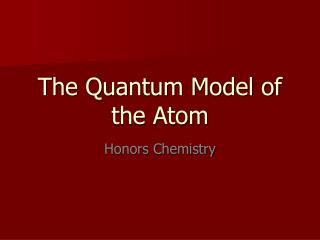Download PresentationThe Quantum Model of the Atom

# The Quantum Model of the Atom - PowerPoint PPT PresentationDownload Presentation## The Quantum Model of the Atom

- - - - - - - - - - - - - - - - - - - - - - - - - - - E N D - - - - - - - - - - - - - - - - - - - - - - - - - - -
##### Presentation Transcript

1. The Quantum Model of the Atom Honors Chemistry

2. Louie de Broglie Proposed that all particles of matter that move exhibit wave like behavior(even a baseball!) He came up with the following equation that relates the wavelength of a particle to its mass and velocity. (Derived from E=mc2 and E = hc/)  = h_ mv Using the mass of an electron moving at the speed of light, he calculated the same energy level values as Bohr did for the Hydrogen atom.

3. Louis de Broglie De Broglie realized that the electrons exhibit wavelike properties in their quantized orbits. (draw pictures ) He said that if an electron has wavelike motion and is restricted to circular orbits of a fixed radius, the electron is allowed only certain possible wavelengths, frequencies and energies. Experiments did show that electrons in atoms do exhibit wave behavior with specific frequencies.

4. Standing wave animation

5. The Heisenberg Uncertainty Principle Heisenberg concluded that it is impossible to make any measurement on an object without disturbing it – at least a little. Electrons are detected by photons and because a photon and an electron have the same energy, any attempt to locate an electron with a photon will knock the electron off course. Therefore: It is impossible to know both the position and the velocity of an electron at the same time. So we can only talk about the probability of finding and electron in certain area (remember the fuzzy cloud!)

6. Erwin Schrodinger Used the idea that electrons behave like waves to write and solve a mathematical equation to describe the location and energy of an electron in the hydrogen atom. The modern description of the electron cloud in atoms comes from the solutions to the Schrodinger equation. This equation showed that the energyof electrons are restricted to certain values.

7. Erwin Schrodinger • However, the equation does not define the exact path the electron takes around the nucleus. • It only estimates the probabilityof finding an electron in a certain position, unlike Bohr’s circular orbits. • Electrons exist in regions called Orbitals • Orbital – 3 dimensional cloud shape around the nucleus that indicates the probable location of an electron

8. Atomic Orbitals The solutions to the Schrodinger equation are called Quantum Numbers, and they are used to describe the properties, such as the energy level and shape (s, p, d or f), and Orientation of the atomic orbitals.

9. “s” - orbital • Each orbital’s shape is determined by it’s the electron’s energy, which is related to its wavelength!!!!!

10. “p” orbitals An Electron in a p orbital has a different wavelength and therefore a different energy. This gives it a different shape!.

11. “d” orbitals

12. Quantum Number Information Definition: Set of 4 numbers that tells us the probable location of the electron. It is like a zip code for the electron The numbers tell us the energy level, shape and orientation of the orbital And, it tells us the spin directionof the electron in that orbital

13. Quantum Number Information Indicates the main energy level occupied by the electron n = 1, 2, 3, . . . 7. . Indicates the shape of the orbital occupied by the electron l = 0 to (n-1) l = 0 “s” orbital l = 1 “p” orbital l = 2 “d” orbital Indicates the orientation of the orbital around the nucleus m = -lto +l If l = 1 Then m = -1, 0 , +1 Indicates the spin direction of the electron. +½ or -½

14. Only 2 electrons are allowed in each orbital Each with an opposite spin. Given the following quantum numbers, describe the “probable” location of the electron. n, l, m, s, 2, 1, 0, + ½ The electron is in energy level 2 It is in a “p” orbital The orientation of the orbital is the y-axis

15. Quantum # and Atomic Structure s 1 1 2 2 s p 1 3 s= 2 p= 6 8 4 s p d 1 3 5 s= 2 p = 6 d = 10 9 18 s p d f 1 3 5 7 s = 2 p = 6 d = 10 f = 14 32 16